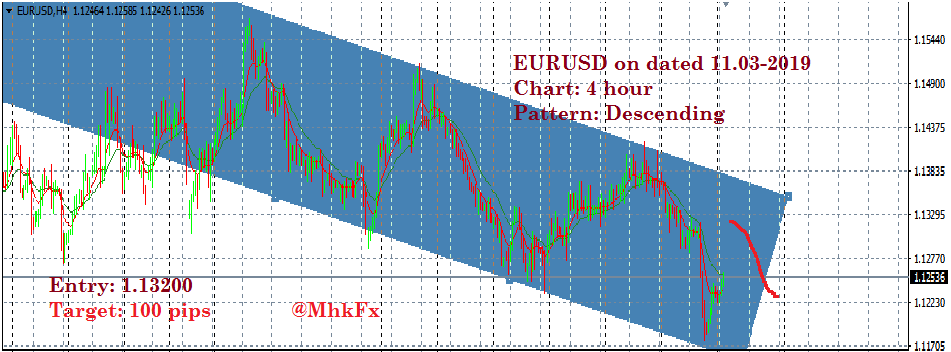694In the context of forex (foreign exchange) trading, a “pip” stands for “percentage in point” or sometimes “price interest point.” It is a standardized unit of measurement used to quantify changes in the exchange rate between two currencies. Pips are typically used to express the smallest price movement that can occur in the exchange rate of a currency pair.

Here are some key points to understand about pips in forex trading:

Definition: A pip is the smallest price move that a given exchange rate can make based on market convention. For most currency pairs, a pip is usually equivalent to 0.0001, or 1/100th of 1%, which is often denoted as 1/10,000th. For example, if the EURUSD currency pair moves from 1.1200 to 1.1201, it has moved up by one pip.

Exception: The Japanese Yen (JPY) is an exception to the typical pip value. For currency pairs involving the JPY, a pip is often defined as 0.01, or 1/100th of 1%. So, if the USDJPY pair moves from 110.00 to 110.01, it has moved up by one pip.

Calculating Profit and Loss: Pips are essential for calculating profit and loss in forex trading. The change in the number of pips between the entry and exit points of a trade determines whether you make a profit or incur a loss. For example, if you buy the EURUSD pair at 1.1200 and sell it at 1.1300, you have gained 100 pips.

Leverage: Many forex traders use leverage, which allows them to control a larger position size with a smaller amount of capital. However, leverage magnifies both potential profits and losses, so understanding pips is crucial for risk management.

Fractional Pips: Some brokers and trading platforms also offer fractional pips, or “pipettes,” to provide traders with more precise pricing information. A fractional pip is typically one-tenth of a standard pip and allows for finer granularity in setting stop-loss and take-profit levels.

Volatility: Currency pairs can experience varying levels of volatility, leading to larger or smaller pip movements. Highly liquid and widely traded pairs like EURUSD often have smaller pip values, while less-traded pairs can have larger pip values.

Spread: The spread is the difference between the bid (selling) and ask (buying) prices for a currency pair. In most cases, the spread is measured in pips, and it represents a cost that traders must overcome to make a profit. The tighter the spread, the lower the cost of trading.

Understanding pips is fundamental for forex traders because it helps them assess risk, set stop-loss and take-profit levels, calculate position sizes, and determine the potential rewards of a trade. It’s essential to have a solid grasp of pip values and how they apply to different currency pairs when engaging in forex trading.